TWiki >Rise Web>TWikiUsers > SeungBum>InvestigationOfVanDerWaalsForce (08 Jun 2006, SeungBum? )

-- SeungBum? - 05 Jun 2006

Investigation of Van der Waals Force

1. INTRODUCTION

It is well known that gecko uses Van der Waals force to attach walls or ceilings. But, what is Van der Waals force?

In 1783, J. D. Van der Waals introduced one correction term to allow for the finite size of molecules and another to allow for intermolecular forces. There forces are induced from electrostatic dipoles between atoms and molecules. Actually, Van der Waals force has been used to represent all intermolecular attractions. However, two types of intermolecular attractions are usually considered as Van der Waals force: dispersion forces and dipole-dipole interactions.

It is true that Van der Waals force is much weaker than strong covalent or ionic bonds. But, in the real world, thin inert contamination films cover most real substrates. So, it is not possible to form covalent or ionic bonds between adhesive systems and the substrates. This is the reason why Van der Waals force dedicates strongly to gecko or most of biological systems adhesive forces.

2. Van der Waals Force: DISPERSION FORCE

Dispersion forces are also known as Van der Waals force or London force, which is named after Fritz London who first suggested how they might arise.

This dispersion force is shown between non-polar molecules. But, attractions are induced from electrical difference in nature. Then, why non-polar molecules have attractions against each other?

Even though non-polar molecules have symmetrical structure to minimize electrical distortion, those electron distributions are not evenly distributed at one instant. This is because of electron mobility; electron moves toward one end of molecules, and it makes molecules polarized transiently. (Fig. 1)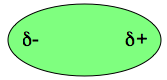Fig. 1: δ+ means slightly positive, δ- means slightly negative.

This transient polarized molecule induces neighbor molecule to be polarized as well, just induced polarity of molecule is reverse. (Fig. 2) Therefore, intermolecular attraction is formed for polarity difference between + and -. This attraction force is called as London dispersion force or Van der Waals force.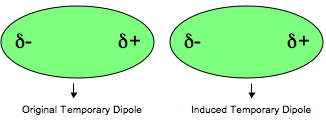Fig. 2: Induced dipole

This transient polarization will continue to fluctuate because of election movement, but induced polarities are synchronized so that the attraction from polarity is maintained as long as the molecules are close together. Below figure shows array of molecules attraction by dispersion force. (Fig. 3)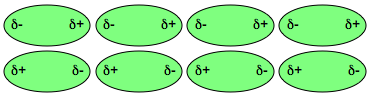Fig. 3: Array of molecules, which have temporary dipole

3. Van der Waals Force: dipole-dipole interactions

A molecule like HCl has a permanent dipole because chlorine is more electronegative than hydrogen; in other words, chlorine attracts electrons strongly rather than hydrogen. This electronegative difference makes electron rich  electron poor region in molecules. And this induces permanent dipole in molecule. From the permanent dipole  dipole interactions, attraction force is formed. (Fig. 4)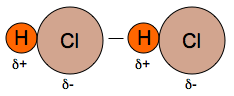Fig. 4: Dipole-dipole interactions

It is important to know that all molecules have dispersion force above mentioned. Dipole  dipole interactions are not alternative to dispersion force; it is just added to dispersion force. Actually, Dipole-dipole interactions are usually minor compared to dispersion force.

For example, the oxygen atoms in CO2 are electron-rich, while the carbon atom in the center is electron poor, so the oxygen atom of one CO2 can be attracted to the carbon of another during very close encounters.

4. Van der Waals Force Strength

Most of interactions between dipoles are based on the Coulombic potential energy of interaction between two charges.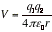(q1, q2: two charges, r: distance between two charges) 

(a) The potential energy of interaction

The potential energy of interaction between a point dipole μ1=q1L and the point charge q2 is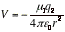(μ in coulomb-meters, q2 in coulombs, r in meters, V in joules) 

A point dipole is a dipole in which the separation between the charges is much smaller than the distance at which the dipole is being observed, L << r.The potential energy rises towards zero more rapidly (as 1/r2 ) than that between two point charges (as 1/r ) because the partial charges of the dipole seem to merge and cancel as the distance r increases.

The various expressions for the interaction of charges and dipoles are summarized in below table.

Interaction Type Distance dependence of potential energy Typical energy/(kJmol-1) Comment
Ion-Ion 1/r 250 Only between ions
Ion-dipole 1/r2 15
Dipole-dipole 1/r3

1/r6
2

0.6
Between stationary polar molecules (solids)

Between rotating polar molecules
London (dispersion) 1/r6 2 Between all types of molecules

The feature to remember is that the interaction energy falls off more rapidly the higher the order of the multi-pole. For the interaction of an n-pole with an m-pole, the potential energy varies with distance as V ∝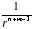(b) Dipole-dipole interactions

The interaction energy of two freely rotating dipoles is zero. However, because their mutual potential energy depends on their relative orientation, the molecules do not in fact rotate completely freely, even in a gas. In fact, the lower energy orientations are marginally favored, so there is a nonzero average interaction between polar molecules.

Average potential energy of two rotating molecules that are separated by a distance r is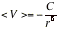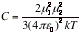At 25C the average interaction energy for pairs of molecules with μ = 1 D is about -0.07 kJ mol-1 (negative sign means the average interaction is attractive) when the separation is 0.5 nm. This energy should be compared with the average molar kinetic energy of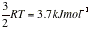at the same temperature.

(The units of dipole moment are generally the Debye (D): 1 D = 3.336 ×10−30C.m)

(c) Dipole-induced dipole interactions

The induced dipole interacts with the permanent dipole of the first molecule, and the two are attracted together. The average interaction energy when the separation of the molecules is r is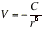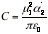(α2 is the polarizability volume of molecule 2 and μ1 is the permanent dipole moment of molecule 1)

For a molecule with μ = 1 D (such as HCl) near a molecule of polarizability volume α=10x10-30m3 (such as benzene), the average interaction energy is about -0.8 kJmol-1 when the separation is 0.3 nm.

(d) Induced-dipole-induced-dipole interactions

This interaction is called either the dispersion interaction or the London interaction. The strength of the dispersion interaction depends on the polarizability of the first molecule because the instantaneous dipole moment depends on the looseness of the control that the nuclear charge exercises over the outer electrons. The strength of the interaction also depends on the polarizability of the second molecule, for that polarizability determines how easily a dipole can be induced by another molecule. Approximation to the interaction energy is given by the London formula: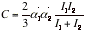(I1 and I2 are the ionization energies of the two molecules)

The dispersion interaction generally dominates all the interactions between molecules other than hydrogen bonds.

(e) The total attractive interaction

The total attractive interaction energy between rotating molecules is the sum of the three Van der Waals contributions discussed above. (Only the dispersion interaction contributes if both molecules are non-polar.)

In a fluid phase, all three distributions to the potential energy vary as the inverse sixth power of the separation of the molecules, so it would be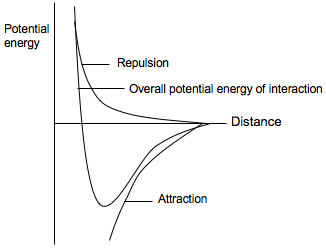The expressions have been derived by assuming that the molecules can rotate freely. That is not the case in most solids, and in rigid media the dipole-dipole interaction is proportional to 1/r3.

Copyright &Â© by the contributing authors. All material on this collaboration platform is the property of the contributing authors.
Ideas, requests, problems regarding TWiki? Send feedback